# Analytical Mathematics lec 2.pptx

29 de May de 2023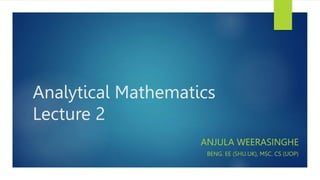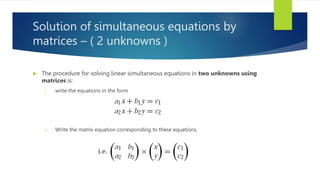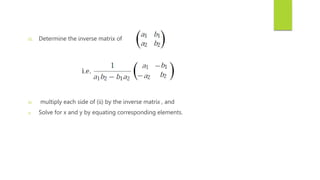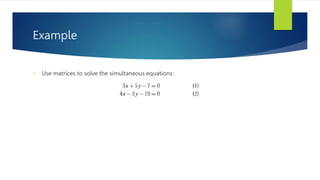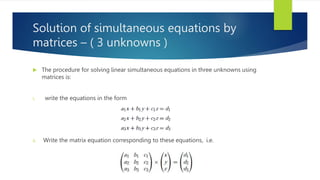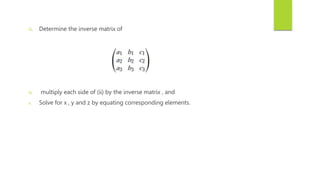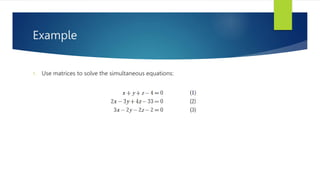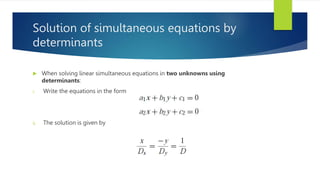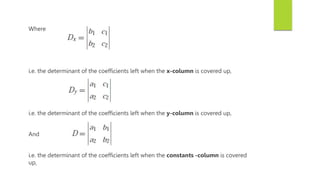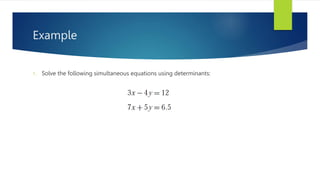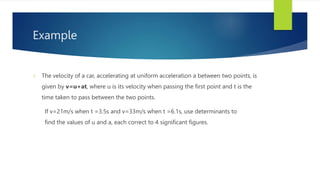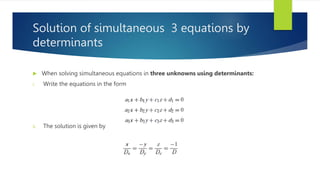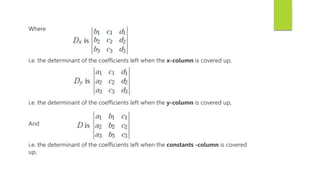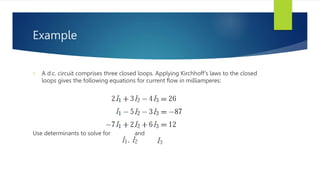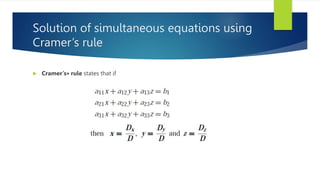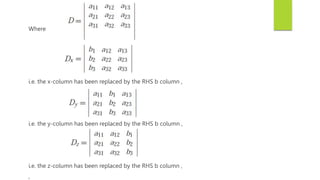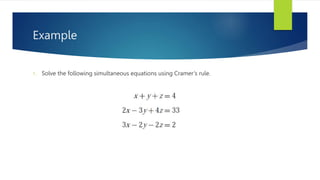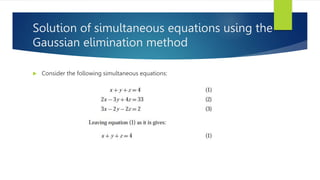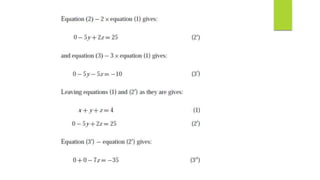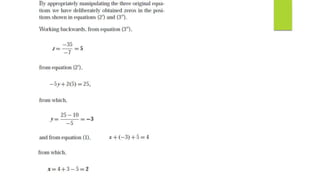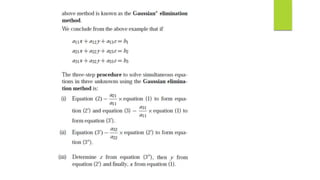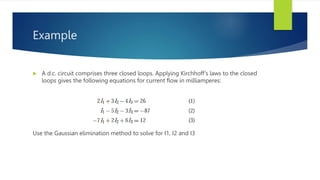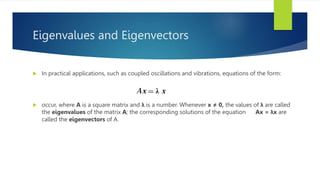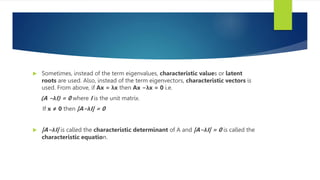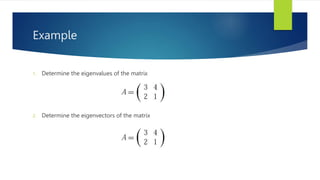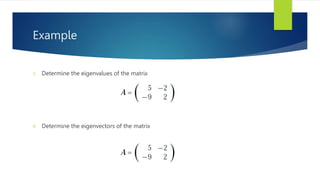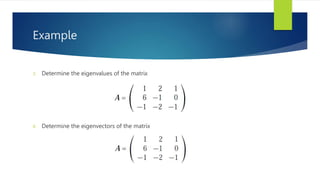1 de 28

### Analytical Mathematics lec 2.pptx

• 1. Analytical Mathematics Lecture 2 ANJULA WEERASINGHE BENG. EE (SHU.UK), MSC. CS (UOP)
• 2. Applications of matrices & determinants
• 3. Solution of simultaneous equations by matrices – ( 2 unknowns )  The procedure for solving linear simultaneous equations in two unknowns using matrices is: i. write the equations in the form ii. Write the matrix equation corresponding to these equations,
• 4. iii. Determine the inverse matrix of iv. multiply each side of (ii) by the inverse matrix , and v. Solve for x and y by equating corresponding elements.
• 5. Example 1. Use matrices to solve the simultaneous equations:
• 6. Solution of simultaneous equations by matrices – ( 3 unknowns )  The procedure for solving linear simultaneous equations in three unknowns using matrices is: i. write the equations in the form ii. Write the matrix equation corresponding to these equations, i.e.
• 7. iii. Determine the inverse matrix of iv. multiply each side of (ii) by the inverse matrix , and v. Solve for x , y and z by equating corresponding elements.
• 8. Example 1. Use matrices to solve the simultaneous equations:
• 9. Solution of simultaneous equations by determinants  When solving linear simultaneous equations in two unknowns using determinants: i. Write the equations in the form ii. The solution is given by
• 10. Where i.e. the determinant of the coefficients left when the x-column is covered up, i.e. the determinant of the coefficients left when the y-column is covered up, And i.e. the determinant of the coefficients left when the constants -column is covered up,
• 11. Example 1. Solve the following simultaneous equations using determinants:
• 12. Example 1. The velocity of a car, accelerating at uniform acceleration a between two points, is given by v=u+at, where u is its velocity when passing the first point and t is the time taken to pass between the two points. If v=21m/s when t =3.5s and v=33m/s when t =6.1s, use determinants to find the values of u and a, each correct to 4 significant figures.
• 13. Solution of simultaneous 3 equations by determinants  When solving simultaneous equations in three unknowns using determinants: i. Write the equations in the form ii. The solution is given by
• 14. Where i.e. the determinant of the coefficients left when the x-column is covered up, i.e. the determinant of the coefficients left when the y-column is covered up, And i.e. the determinant of the coefficients left when the constants -column is covered up,
• 15. Example 1. A d.c. circuit comprises three closed loops. Applying Kirchhoff’s laws to the closed loops gives the following equations for current flow in milliamperes: Use determinants to solve for and
• 16. Solution of simultaneous equations using Cramer’s rule  Cramer’s∗ rule states that if
• 17. Where i.e. the x-column has been replaced by the RHS b column , i.e. the y-column has been replaced by the RHS b column , i.e. the z-column has been replaced by the RHS b column , ,
• 18. Example 1. Solve the following simultaneous equations using Cramer’s rule.
• 19. Solution of simultaneous equations using the Gaussian elimination method  Consider the following simultaneous equations:
• 23. Example  A d.c. circuit comprises three closed loops. Applying Kirchhoff’s laws to the closed loops gives the following equations for current flow in milliamperes: Use the Gaussian elimination method to solve for I1, I2 and I3
• 24. Eigenvalues and Eigenvectors  In practical applications, such as coupled oscillations and vibrations, equations of the form:  occur, where A is a square matrix and λ is a number. Whenever x ≠ 0, the values of λ are called the eigenvalues of the matrix A; the corresponding solutions of the equation Ax = λx are called the eigenvectors of A.
• 25.  Sometimes, instead of the term eigenvalues, characteristic values or latent roots are used. Also, instead of the term eigenvectors, characteristic vectors is used. From above, if Ax = λx then Ax −λx = 0 i.e. (A −λI) = 0 where I is the unit matrix. If x ≠ 0 then |A−λI| = 0  |A−λI| is called the characteristic determinant of A and |A−λI| = 0 is called the characteristic equation.
• 26. Example 1. Determine the eigenvalues of the matrix 2. Determine the eigenvectors of the matrix
• 27. Example 3. Determine the eigenvalues of the matrix 4. Determine the eigenvectors of the matrix
• 28. Example 3. Determine the eigenvalues of the matrix 4. Determine the eigenvectors of the matrix